# Cross sections

### Purely leptonic reactions: calculated total cross sectionsNeutrinos interact only by the weak force: hence the reaction cross sections are much smaller than those of other particle species. For electron neutrinos the elastic scattering with electrons can be mediated by charged and neutral weak bosons (W and Z), whereas the scattering of muon and tau neutrinos from electrons is mediated only by the neutral boson. Hence the differences of the total cross sections.

The inelastic scattering processes are caused only by the charged currents.

The cross section formulae for tau neutrinos are the same as for muon neutrinos.

Elastic scattering Inelastic scattering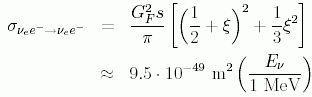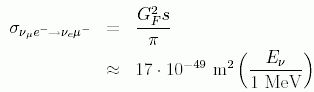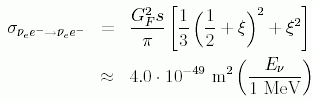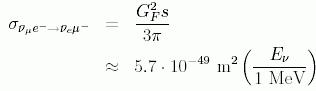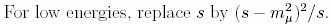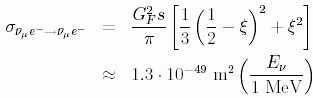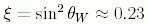### Neutrino reactions with nucleons and nuclei

The cross sections for neutrino-nucleon scatterings are not so precisely known as for leptonic reactions. This is due to the poor theoretical knowledge of the nucleon form factors.

Neutrino-nucleon inelastic cross sections:

For energies < 1 GeV: At low energies the neutrino-nucleon cross sections acquire the following form, where Q = 1.3 MeV is the nucleon mass difference and theta(E-Q) is just the threshold function:

Neutrino-nucleon Antineutrino-nucleon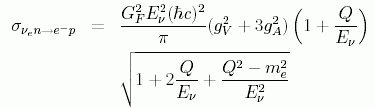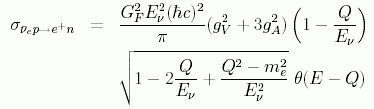The vector and axial-vector coefficients have values g_V = 1 and g_A = 1.23.
Hence the multiplier in the equations has the value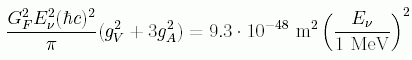For very high energies (50 GeV < E < 250 GeV) the neutrino-nucleon inclusive cross sections are approximately:

Neutrino-nucleon Antineutrino-nucleon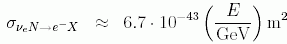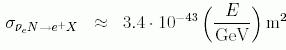These approximate results are also valid for muon and tau -neutrinos at high energies.

For energies around 1...10 GeV the cross sections are not so accurately known:
Below is a plot of different experimental results for the muon and antimuon -neutrino total cross sections. (Taken from K. Hagiwara et al., Phys. Rev. D 66 (Review of Particle Physics 2002))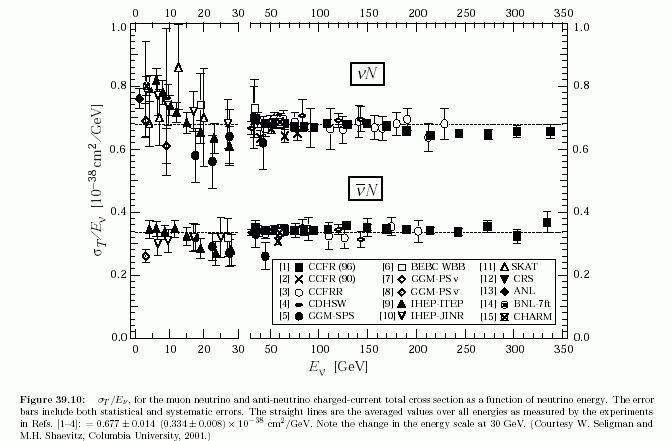Neutrino-nucleon elastic total cross sections:

Neutrino-nucleon elastic c.s.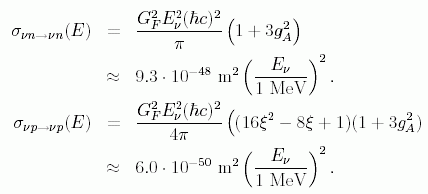These are valid for all neutrino species.

For more detailed cross section information (differential cross sections, etc.) see Appendix chapter 4.3.2.

Back to mainpage